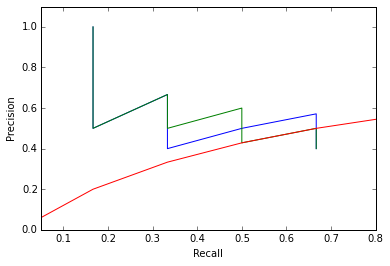## Lecture 8: evaluating ranked retrieval systems¶

We will discuss some measures for evaluating rank retrieval methods.

To start let's imagine we have an information need that is satisfied by a set Relevant of relevant documents and three rankings returned by three systems. We want a measure that tells us which one provides a better ranking. Note that what we mean by "better" can depend on the use case.

In :
from __future__ import print_function
from __future__ import division

%matplotlib inline
import matplotlib.pyplot as plt

In :
## 6 relevant documents:
Relevant=["A","B","C","D","E","F"]

## rankings returned by 3 systems, we use # for non=relevant results:
S1=["A",
"#",
"B",
"#",
"#",
"C",
"D",
"#",
"#",
"#"]

S2=["A",
"#",
"B",
"#",
"C",
"#",
"#",
"D",
"#",
"#"]

S3=["#",
"#",
"#",
"#",
"A",
"B",
"C",
"D",
"E",
"F"]


calculating set precision and recall

In :
def isRelevant(item):
if item in Relevant:
return True

def precision(Sys):
return len([item for item in Sys if isRelevant(item)])/len(Sys)

def recall(Sys):
return len([item for item in Sys if isRelevant(item)])/len(Relevant)


since precision is a set measure, it should be the same for S1 and S2 should be the same (they return the same results)

In :
print(precision(S1))
print(precision(S2))
print(precision(S3))

0.4
0.4
0.6


same for recall:

In :
print(recall(S1))
print(recall(S2))
print(recall(S3))

0.666666666667
0.666666666667
1.0


the first attempt to take the ranking into account is to calculate precision@k: the precision of the set formed by the first k returned documents

In :
def precision_at_k(Sys,k):
return precision(Sys[:k])

print (" ".join([str("Rank"), str("Item"), "P@k"]))
for rank,item in enumerate(S1):
rank+=1
print ("    ".join([str(rank), str(item), "{0:.2f}".format(precision_at_k(S1,rank))]))

Rank Item P@k
1    A    1.00
2    #    0.50
3    B    0.67
4    #    0.50
5    #    0.40
6    C    0.50
7    D    0.57
8    #    0.50
9    #    0.44
10    #    0.40

In :
print (" ".join([str("Rank"), str("Item"), "P@k"]))
for rank,item in enumerate(S2):
rank+=1
print ("    ".join([str(rank), str(item), "{0:.2f}".format(precision_at_k(S2,rank))]))

Rank Item P@k
1    A    1.00
2    #    0.50
3    B    0.67
4    #    0.50
5    C    0.60
6    #    0.50
7    #    0.43
8    D    0.50
9    #    0.44
10    #    0.40


We can do the same for recall

In :
def recall_at_k(Sys,k):
return recall(Sys[:k])

print (" ".join([str("Rank"), str("Item"), " P@k","   R@k"]))
for rank,item in enumerate(S1):
rank+=1
print ("    ".join([str(rank), str(item), "{0:.2f}".format(precision_at_k(S1,rank)),"{0:.2f}".format(recall_at_k(S1,rank))]))

Rank Item  P@k    R@k
1    A    1.00    0.17
2    #    0.50    0.17
3    B    0.67    0.33
4    #    0.50    0.33
5    #    0.40    0.33
6    C    0.50    0.50
7    D    0.57    0.67
8    #    0.50    0.67
9    #    0.44    0.67
10    #    0.40    0.67


And now we print the precision vs recall, i.e., the "precision-recall" plot

In :
plt.plot([recall_at_k(S1,rank) for rank in range(1,11)],[precision_at_k(S1,rank) for rank in range(1,11)])
plt.plot([recall_at_k(S2,rank) for rank in range(1,11)],[precision_at_k(S2,rank) for rank in range(1,11)])
plt.plot([recall_at_k(S3,rank) for rank in range(1,11)],[precision_at_k(S3,rank) for rank in range(1,11)])

plt.xlabel("Recall")
plt.ylabel("Precision")
plt.xlim([0.05,0.8])
plt.ylim([0,1.1])

Out:
(0, 1.1)We can see that S3 stands out as a system that favore recall and sacrifces precision to the top. However, we might still want to summarize the performance in a single number.

One way to do this is to calculate "Average precision". This measure averages precisions calculated every time a new relevant document is retrieved.